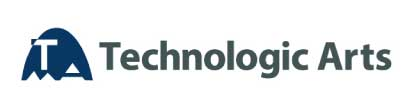#Language： 日本語 中文# UML Training Series

## Introduction to UML

Outline You will learn the basics of modeling by using each diagram and comprehend the notation UML2　that will focus on the on-the-spot diagrams while practicing with exercises. To comprehend the whole picture such as positioning, significance of UML To understand the meaning and notation with respect to the diagrams which are commonly used For engineers who would like to learn the basics of modeling and notation of UML2 1 day Be familiar with common software development Overview of modeling and UML UML diagrams Basic knowledge of object-oriented Details of each diagram Use case diagram Activity diagram Object diagram Class diagram Sequence diagram State Machine Diagram Layout drawing Common element Relations between diagrams ¥41,040 ( tax included ) / person 8

## Countermeasures for UML Modeling Skill Certification Test L1 (T1, T2)

Outline You will acquire UMTP/UML modeling skill within the scope of UMTP/UML Modeling Skill Authorized Examination at Level 1(T1, T2) while practicing by using the text that is based on the UMTP standard. To acquire the basic knowledge of modeling by using UML and enhance the skills of taking UML Modeling Skill Authorized Examination at Level 1. For those who are willing to take the exam of UML Modeling Skills Authorized Exam Level 1 and acquire the basic knowledge of UML 1 day Basic knowledge of object-oriented Basic knowledge development process , modeling Request modeling ( use case diagram ) Structural modeling ( class diagram ) Behavior modeling ( sequence diagram ) Behavior modeling ( collaboration diagram ) Behavior modeling ( state diagram ) Behavior modeling ( activity diagram ) Implementation modeling ( component view) Implementation modeling ( layout drawing ) Common element Modeling by using multiple diagrams ¥41,040 ( tax included ) / person 8

## Practice UML Modeling

Outline You will practically learn “Component-based modeling” which is focused on the “parts” that is for securing maintainability and reusability. Also, it will be focused on “Business Process Modeling” from the upper process, acquire the basic skills to support EA, SOX method and SOA etc. To comprehend the flow of component-based modeling by using UML, get the hang of modeling of each process (particle size, details degree etc.) as well as the modeling method that ensures the seamless resistance from upper to lower process. For engineers who would like to practice the analysis and design by using UML For engineers who would like to acquire skills of software development with high maintainability and reusability 2 days What is component-based development What is UML What is MDA ( model-driven development ) Modeling Practice (from upper to lower process) General exercises ¥82,080 ( tax included ) / person 8

## Introduction to SysML

Outline You will learn System Modeling Language (SysML) from its outline to notation of each diagram. Also it will help you have a better understanding on describing the diagram by practicing with tools. To gain the basic knowledge of SysML For engineers who want to get the basic knowledge of SysML 1 day Overview of SysML Introduction of elements and each diagram Flow of SysML modeling Exercise: Notation of each diagram Sample of the diagram ¥41,040 ( tax included ) / person 8